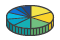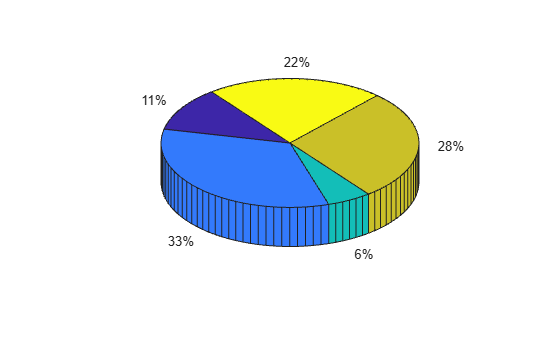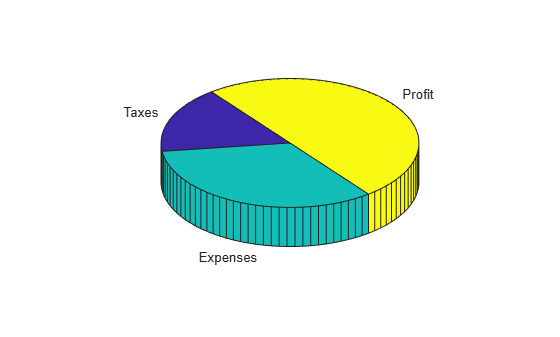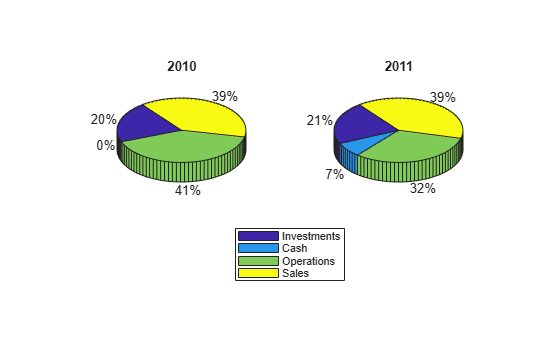# pie3

•## Syntax

```pie3(X) pie3(X,explode) pie3(...,labels) pie3(axes_handle,...) h = pie3(...) ```

## Description

`pie3(X)` draws a three-dimensional pie chart using the data in `X`. Each element in `X` is represented as a slice in the pie chart.

• If `sum(X) ≤ 1`, then the values in `X` directly specify the area of the pie slices. `pie3` draws only a partial pie if ```sum(X) < 1```.

• If the sum of the elements in `X` is greater than one, then `pie3` normalizes the values by `X/sum(X)` to determine the area of each slice of the pie.

`pie3(X,explode)` specifies whether to offset a slice from the center of the pie chart. `X(i,j)` is offset from the center of the pie chart if `explode(i,j)` is nonzero. `explode` must be the same size as `X`.

`pie3(...,labels)` specifies text labels for the slices. The number of labels must equal the number of elements in `X`.

`pie3(axes_handle,...)` plots into the axes with the handle `axes_handle` instead of into the current axes (`gca`).

`h = pie3(...)` returns a vector of handles to patch, surface, and text graphics objects.

## Examples

collapse all

Create a 3-D pie chart of vector `x`.

```x = [1,3,0.5,2.5,2]; figure pie3(x)```To offset the second pie slice, set the corresponding `explode` element to 1.

```explode = [0,1,0,0,0]; figure pie3(x,explode)```Create a 3-D pie chart and specify the text labels.

```x = 1:3; labels = {'Taxes','Expenses','Profit'}; figure pie3(x,labels)```Create vectors `y2010` and `y2011`, that contain financial data for two years. Then create a cell array containing the labels for the values.

```y2010 = [50 0 100 95]; y2011 = [65 22 97 120]; labels = {'Investments','Cash','Operations','Sales'};```

Create a `2`-by-`1` tiled chart layout, and display a pie chart and legend for `y2010` data in the first tile. Then display a pie chart and legend for the `y2011` data in the second tile.

```t = tiledlayout (2,1); ax1 = nexttile; pie3(ax1,y2010) title('2010') legend(labels) ax2 = nexttile; pie3(ax2,y2011) title('2011') legend(labels)```## Compatibility Considerations

expand all

Behavior changed in R2019b